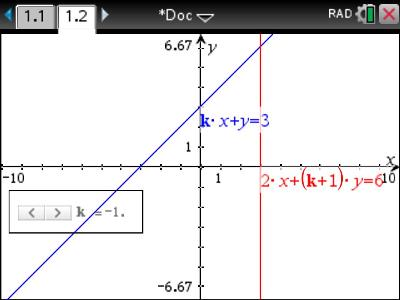# Activities

•• ##### Subject Area

• Standard: VCE: Mathematical Methods: Mathematical Methods

• ##### AuthorAust Senior

60 Minutes

• ##### Device
•TI-Nspire™ CX CAS
• TI-Nspire™ Apps for iPad®
• ##### Software

TI-Nspire™ CAS
TI-Nspire™ CAS Navigator™ NC System

4.4

## Moveable Lines#### Activity Overview

Finding solutions to two linear simultaneous equations is straight forward, until someone puts a parameter in it! Including parameters changes the complexity of the question, so using a slider to provide a visualisation of the problem can make things a lot easier to understand.

#### Objectives

• General solution of equations involving a single parameter

• Linear
• Infinite
• Parameter
• Parallel

#### About the Lesson

Students are provided with two sets of linear simultaneous equations each containing a parameter. A slider is automatically created when the graphs are produced so that students can explore how the parameter effects the interaction of the two graphs, students then work on the interpretation of the algebraic solutions.